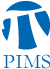## PIMS-UW Mathematics Colloquium: S. R. Srinivasa Varadhan

• Date: 05/10/2013
• Time: 14:30
Lecturer(s):

S. R. Srinivasa Varadhan, Courant Institute, NYU
Location:

University of Washington

Topic:

Let H_i, i=1,2,...,k be a finite collection of finite graphs. Let v_i be the number of vertices in H_i. We want to count the number of graphs G with N vertices in which Hi appears roughly c_i N v_i times. The tools are a combination of large deviations in probability theory and Szemeredi's regularity theorem for dense graphs.

Description:

UW-PIMS Mathematics Colloquium

Schedule:

May 10, 2013 at 2:30pm in DEM 004